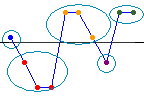# Specify the comparison criterion for Runs Test

Stat > Nonparametrics > Runs Test
Select the value that Minitab uses to calculate the number of runs.A run is a group of consecutive data points above or below the comparison criterion, K. The line represents K, which gives this example five runs.
• Above and below the mean: Calculate the number of runs that are above and below the mean. Usually, using the mean is better than specifying a value in Above and below.
• Above and below: Calculate the number of runs that are above and below the value that you specify. If the data contain extreme values, using the median to minimize the effect of the extreme values may be more appropriate.